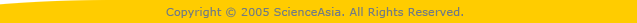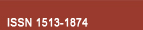| Home  | About ScienceAsia  | Publication charge  | Advertise with us  | Subscription for printed version  | Contact us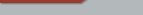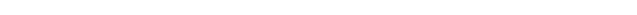Editorial Board Journal Policy Instructions for Authors Online submission Author Login Reviewer Login Volume 49 Number 5 Volume 49 Number 4 Volume 49 Number 3 Volume 49 Number 2 Volume 49 Number 1 Volume 48 Number 6 Earlier issues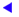Volume 44 Number 6Volume 45 Number 1 Volume 45 Number 2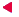previous article next article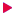9554

Research articles

ScienceAsia 45 (2019): 74-84 |doi: 10.2306/scienceasia1513-1874.2019.45.074

# Convergence analysis of three parareal solvers for impulsive differential equations

## Zhiyong Wanga, Liping Zhangb,*

ABSTRACT:     We are interested in using the parareal algorithm consisting of two propagators, the fine propagator F and the coarse propagator G, to solve the linear differential equations u(t)+Au(t) = ƒ with stable impulsive perturbations Δu(t) = αu(t-) for t = τl, where α ∈ (-2,0), Δu(t) = u(t+) ? u(t-), and I ∈ N. We consider the case that A is a symmetric positive definite matrix and G is defined by the implicit Euler method. In this case, provable results show that the algorithm possesses constant convergence factor ? ≈ 0.3 if α = 0 and F is an L-stable numerical method. However, if F is not L-stable, such as the widely used Trapezoidal rule, it unfortunately holds that ? ≈ 1 if λmax>>1, where λmax is the maximal eigenvalue of A. We show that with stable impulses the parareal algorithm possesses constant convergence factors for both the L-stable and A-stable F-propagators, such as the implicit Euler method, the Trapezoidal rule and the 4th-order Gauss Runge-Kutta method. Sharp dependence of the convergence factor of the resulting three parareal algorithms on the impulsive parameter α is derived and numerical results are provided to validate the theoretical analysis.